# Percent to number

To increase that number by 5 percent, we need to multiply it by:

x =  1.05

### Step-by-step explanation:

$x=1+\frac{5}{100}=\frac{21}{20}=1\frac{1}{20}=1.05$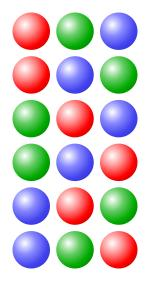Did you find an error or inaccuracy? Feel free to write us. Thank you!Tips to related online calculators

## Related math problems and questions:

• Percentage increaseIncrease number 400 by 3.5%
• Percentages and numbers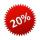How many percent is number 69 smaller than number 276?
• Positive number z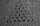Positive number z is 10% greater than the number y. How many % is y smaller than z? Report the result rounded to one decimal place.
• The number 72The number 72 increase by 25%. By how much % will you have to reduce the number you created to get the number 72 again?
• If weIf we increase the unknown number by 4%, we get 780. Determine the unknown number.
• Four numbersThe first number is 50% second, the second number is 40% third, the third number is 20% of the fourth. The sum is 396. What are the numbers?
• Price increase 2xIf two consecutive times we increase the price of the product by 20%, how many % is higher final price than the original?
• 3 years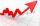The company has increased the number of employees. In year 2005 compared to 2004 13% more, in 2006 18% more against the previous year, in year 2007 20% more compared to 2006. How many % increase in the number of employees in 3 years?
• The fastestThe fastest boat can reach speeds more than 710% as fast as the Queen Mary 2. How would you express this number as a fraction and as a decimal?
• Percentages above 100%What is 122% of 185? What is the meaning of percentages above 100%?
• Say we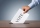Say we have 70 voting delegates and we need a 2/3 vote What would that number be? Also same as above but in this virtual world, it converts to percentages. What would the % number of positive votes be?
• Two-digit numberIn a two-digit number, the number of tens is three greater than the number of units. If we multiply the original number by a number written in the same digits but in reverse order, we get product 3 478. Find the original number.
• Sum of three numbersThe sum of three numbers from which the second number is 20% smaller than the first number and the third number is 25% smaller than the second number is 96. Determine these numbers.
• PercentCalculate how many % is the number 26.25 less than the number 105.
• Unknown number 10Number first increased by 30%, then by 1/5. What percentage we've increased the original number?
• Unknown number 5I think of an unknown number. If we enlarge it five times, then subtract 3, and the result decreases by 75%, we get one greater than the number. What number am I thinking of?
• The ratio 4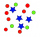The ratio of two number is 5:4 if 40% of the first number is 12, what will be 50% of the second number?